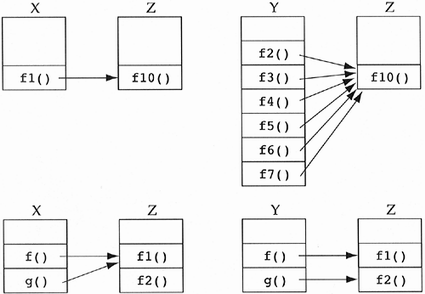### 4.5 Refining the Amount of Collaboration Between Two Classes

From the viewpoint of logical design, once a collaboration exists between two classes, a certain level of complexity has been added to the system. It does not matter whether two different messages are sent between the two classes or one hundred messages are sent. The complexity is constant. If we view the system from its implementation standpoint (e.g., physical design), we find some other interesting forms of complexity. Consider these questions and their associated diagrams in Figure 4.9.

##### Figure 4.9. Additional collaboration complexities: which designs are more complex?1. What is the effect on the complexity of a system if a class has many message sends to a collaborating class instead of just one? For example, how do we compare the complexity of a class X, which has a method f1() that sends a class Z an f10() message, to a class Y, which has six methods f2(), f3(), f4(), f5(), f6(), and f7(), each of which sends Z the f10() message? At logical design time we simply state that X uses Z and Y uses Z. Intuitively, the class Y seems more complex because more of its implementation is tied to Z. If there is a problem with the f10() method of Z, then will more code need to be examined in the case of class Y? Since we do not know the relative complexities of f1() to f2(), f3(), etc., we cannot readily answer what initially seems intuitive.

2. What is the effect on the complexity of a system if a class sends different messages as opposed to the same message? For example, a class X sends the class Z the f1() message from two of its methods versus class Y, which sends Z the f1() and f2() messages each once. Both examples involve two message sends, but we only state the generic "X uses Z and Y uses Z" at logical design time. Again, class Y intuitively seems more complex because it is dependent on two methods of Z instead of just one. We could say that Y collaborates more with Z than does X. We certainly wish to minimize the amount of collaboration in addition to the number of collaborators.

3. What about limiting the amount of fanout that a class exhibits, that is, the product of the number of methods and the messages they send? If a class has seven methods, each of which sends out 10 messages, then that class has a fanout of 70. Should we limit this value? Again, we can certainly see that complexity increases with fanout.

The moral of the story is that each of these examples/questions adds complexity to the design, but they are small correction factors compared to the original concern of too many collaborating classes. We should minimize the number of message sends between two collaborating classes, the number of different ways the classes collaborate, and class fanout. I do not believe we can construct quantitative heuristics to deal with these issues, although several authors have offered them. Heuristics that state "facts," like "fanout should be limited to 50," give little rational explanation for the magic number 50. There is certainly a difference in complexity between a class X that has 2 methods, each of which sends 25 messages to the class Z, and a class Y that has 10 methods, each of which sends 5 messages to the class Z. Why not limit fanout to 45 or 55 or 70? I believe that the qualitative metrics are best provided with no guess as to their quantification.

#### Heuristic 4.2

Minimize the number of message sends between a class and its collaborator.

#### Heuristic 4.3

Minimize the amount of collaboration between a class and its collaborator, that is, the number of different messages sent.

#### Heuristic 4.4

Minimize fanout in a class, that is, the product of the number of messages defined by the class and the messages they send.

If these heuristics are considered equal to the collaboration minimization heuristic (Heuristic 4.1), then we would be implying that there is some break-even point between adding more message sends between classes and adding a new collaboration. For example, we might argue that rather than letting a method of the person class use a new alarm clock message, it might be better for the person class to collaborate with some entirely new class. This is never true, because the big hit in complexity for the uses relationship comes at the class level, not the object level. The fact that the person class is dependent on two classes rather than one is the overriding concern. The amount of coupling between the objects of a class is a much smaller problem.